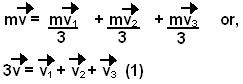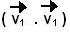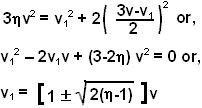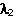## Tuesday, June 3, 2008

### Irodov Problem 1.171

The first principle to use is that momentum of the system just before and after the breaking of the mass will be conserved. If v1, v2 and v3 are the velocity vectors of the three pieces after the breakup, we have,The energy of the system will increases by a factorand so we have,Our goal is to maximize the magnitude of the velocity of one of the pieces. Let us choose to maximize the magnitude of piece 1. So our goal is to maximizewhile satisfying the vector equations given by (1) and (2).

Now we can either solve this rigorously and mathematically in long way or use some intuitive reasoning and common sense to solve it quickly. I will do this both ways.

The Simple WayFirst ask yourself in what directions should the two other pieces move so that piece 1 attains the maximum velocity. Initially the momentum of the composite piece was mv and this must be conserved. Suppose that the two pieces had zero velocity right after the breakup then piece 1 would have to move at 3v in order to conserve the original momentum of the mass. Can we do better? Ofcourse!! if the other pieces shot out in the opposite direction at velocity v' each as shown in the figure then,In principle this is not very different from a rocket where the fuel shoots of backwards pushing the rocket forward. From equation (2) and (3) we have,Neglecting the negative sign we have.

Solving it the hard way
The problem is an optimization problem where we have to maximizesubject to constraints given by (1) and (2). We can use Lagrangian multipliers to solve the problem. We create the objective function,Sinceis a scalar, what (9) means is that all three vectors v1, v2 and v3 must be collinear!! From constraint (1) it is also clear then that v1, v2, v3 and v must all be collinear. So in essence if we choose x-axis to align with as the direction of motion of the original mass then it becomes exactly like the figure above. So with all the math we have simply arrived at the intuition that we started with in the simple way!! From here, we can follow the simple solution above to obtain the answer.